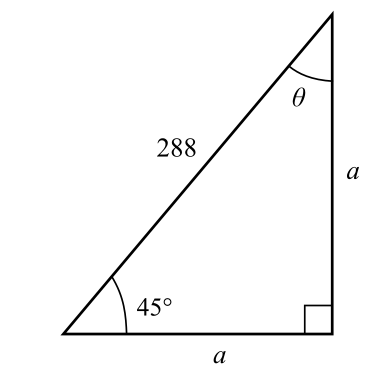Chapter 8.1, Problem 34E### Calculus: An Applied Approach (Min...

10th Edition
Ron Larson
ISBN: 9781305860919

#### Solutions

Chapter
Section### Calculus: An Applied Approach (Min...

10th Edition
Ron Larson
ISBN: 9781305860919
Textbook Problem
1 views

# Analyzing Triangles In Exercises 33-40, solve the triangle for the indicated side and/or angle.To determine

To calculate: The value of side a and angle θ in a triangle provided below.Explanation

Given Information:

The provided triangle is shown below,

Formula used:

In a right triangle, the sum of two acute angles is 90°.

Pythagorean theorem for any right triangle is such that c2=a2+b2, where a, b are the two perpendicular sides of a triangle and c is the length of hypotenuse.

Calculation:

Consider the sides of triangle, a, a and 288

Use the Pythagorean theorem a2+b2=c2.

a2+a2=28822a2=82,

### Still sussing out bartleby?

Check out a sample textbook solution.

See a sample solution

#### The Solution to Your Study Problems

Bartleby provides explanations to thousands of textbook problems written by our experts, many with advanced degrees!

Get Started

#### In problems 31-36, simplify each expression. 36.

Mathematical Applications for the Management, Life, and Social Sciences

#### True or False: f(x) = 3x x3 is concave down for x 1.

Study Guide for Stewart's Single Variable Calculus: Early Transcendentals, 8th

#### Using for |x| < 1,

Study Guide for Stewart's Multivariable Calculus, 8th